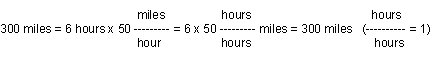# National Aeronautics and Space Administration

## Shedding a New Light on the Universe

### Frequency, Wavelength & Energy Activity

By J. Allie Hajian

### 1. Unit Analysis

Sometimes you want to express a measurement in different units. For example, when talking about how far away something is, sometimes it may be useful to say it is a certain DISTANCE (New York is 300 miles from here), and sometimes it is more useful to use TIME to express how far away it is (New York is a 6 hour drive from here). Of course miles are not equal to hours, so there must be some way to convert from one to the other. In this case, the conversion is speed: if a car drives an average of 50 miles/hour, then it can drive 300 miles in 6 hours. For this constant speed, 300 miles equals 6 hours.Problem

1. If you walk at a speed of four miles an hour, and your friend lives two miles away, how far away is her house
a. in miles
b. in minutes, if you are walking
c. in minutes, if you are driving at an average speed of 25 miles an hour

In much the same way, different units can be used to characterize light. We can refer to light by its wavelength, its frequency, or its energy. This is similar to talking about distance in units of miles or hours.

### 2. Wavelength --> Frequency

Light waves travel at a constant speed. Because of this there is a one to one relationship between light's wavelength and its frequency. If waves are short, there must be more of them in a set amount of time to travel the same distance in that time (the same speed).

Problems

1. The speed of light is 186,000 miles per second. What is the frequency of light that has a wavelength of three feet? two inches? 1/1,000,000 inches? one mile?

2. What is the wavelength of the radio waves of your favorite radio station? (HINT: the frequency of radio stations is equal to the station number times 1,000,000 Hz. So WAMU - National Public Radio - at FM 88.5 - is 88,500,000 Hz. Now, use the fact that the wavelength is equal to the speed of light, a constant, divided by frequency.)

### 3. Frequency --> Energy

In 1900, Planck discovered that there was a direct relationship between a photon's frequency and its energy:

E = h nu

The higher the frequency of light, the higher its energy. We know from the problems above that higher frequencies mean shorter wavelengths. We can also say that E = h c / lambda. High frequency light has short wavelengths and high energy. X-rays or gamma-rays are examples of this. Radio waves are examples of light with a long wavelength, low frequency, and low energy.

In much the same way, the gallons of gas you put in your car and the cost of the gas are proportional: the same value multiplied by a constant (the price of a gallon of gas). If you know the constant (the price per gallon) and you know the number of gallons, you can calculate how much the gas costs. Or, if you know how much the gas cost, you can calculate how much gas was bought.

Problems

1. Planck's constant is 4.136 x 10-15 eV sec. What is the frequency of light that has an energy of 12.5 keV? (Hint: 1 keV = 1000 eV)

2. What is the energy corresponding to the frequency of your favorite radio station? (see problem 3 for the frequency of your favorite radio station). How does that compare to the energy given off by a 50 Watt light bulb in an hour?